# Class 6 Maths NCERT Solutions for Chapter 11 Algebra Ex – 11.1

## Algebra

Question 1.
Find the rule, which gives the number of matchsticks required to make the following matchstick patterns. Use a variable to write the rule.

(a) A pattern of letter T as

(b) A pattern of letter Z as

(c)
A pattern of letter U as

(d)
A pattern of letter V as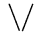(e) A pattern of letter E as

(f)
A pattern of letter S as

(g)
A pattern of letter A asSolution:
(a) For T: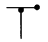Clearly, to make one T, we use 2 matchsticks as shown in the figure. Rule is given as
“Number of matchsticks required = 2n”, where n can take any value 1, 2, 3, …
(b) For Z :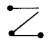Clearly, to make one Z, we use 3 matchsticks as shown in the figure. Rule is given as
“Number of matchsticks required = 3 n”, where n can take any value 1, 2, 3, …
(c) For U :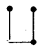Clearly, to make one U, we use 3 matchsticks as shown in the figure. Rule is given as
“Number of matchsticks required = 3n”, where rt can take any value 1, 2, 3, …
(d) For V :Clearly, to make one V, we use 2 matchsticks as shown in the figure. Rule is given as
“Number of matchsticks required = 2n”, where n can take any value 1, 2,3,…
(e) For E :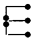Clearly, to make one E, we use 5 matchsticks as shown in the figure. Rule is given as
“Number of matchsticks required = 5n”, where n can take any value 1, 2, 3, …
(f) For S :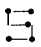Clearly, to make one S, we use 5 matchsticks as shown in the figure. Rule is given as
“Number of matchsticks required = 5n”, where n can take any value 1, 2, 3, …
(g) For A :Clearly, to make one A, we use 6 matchsticks as shown in the figure. Rule is given as
“Number of matchsticks required = 6 n”, where n can take any value 1, 2, 3, …

Question 2.
We already know the rule for the pattern of letters L, C and F. Some of the letters from Q. 1 (given above) give us the same rule as that given by L. Which are these? Why does this happen?

Solution:
(a) and (d) parts of Q. 1. give the same rule as for L. It happens as the same number of matchsticks are being used in these cases.

Question 3.
Cadets are marching in a parade. There are 5 cadets in a row. What is the rule, which gives the number of cadets, given the number of rows? (Use n for the number of rows.)

Solution:
Since, there are 5 cadets in a row and number of rows are n
∴ Rule is given as

Question 4.
If there are 50 mangoes in a box, how will you write the total number of mangoes in terms of the number of boxes? (Use b for the number of boxes.)

Solution:
Since, there are 50 mangoes in a box and b is the number of boxes.
∴ Total number of mangoes = 50b

Question 5.
The teacher distributes 5 pencils per student. Can you tell how many pencils are needed, given the number of students? (Use s for the number of students.)

Solution:
Since, there are s number of students and each student having 5 pencils.
∴ Total number of pencils needed = 5n

Question 6.
A bird flies 1 kilometre in one minute. Can you express the distance covered by the bird in terms of its flying time in minutes? (Use t for flying time in minutes.)

Solution:
Since, a bird flies at 1 kilometre in one minute. If it flies for t minutes. Total distance covered by bird in t minutes = 1 x t km = t km

Question 7.
Radha is drawing a dot Rangoli (a beautiful pattern of lines joining dots with chalk powder. She has 9 dots in a row. How many dots will her Rangoli have for r rows? How many dots are there if there are 8 rows? If there are 10 rows?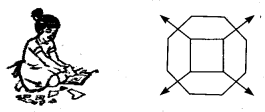Solution:
Since, a row contains 9 dots.
Therefore, number of dots in r rows = 9 r.
Number of dots in 8 rows = 9 x 8 = 72
and, number of dots in 10 rows = 9 x 10 = 90

Question 8.
Leela is Radha’s younger sister. Leela is 4 years younger than Radha. Can you write Leela’s age in terms of Radha’s age? Take Radha’s age to be x years.

Solution:
Let Radha’a age be x years. Since Radha’s younger sister Leela is 4 years younger than Radha.
∴ Leela’s age = (x – 4) year

Question 9.

Solution:
Number of laddus given to guests and family members = l
Number of laddus left over = 5

Question 10.
Oranges are to be transferred from larger boxes into smaller boxes. When a large box is emptied, the oranges from it fill two smaller boxes and still 10 oranges remain outside. If the number of oranges in a small box are taken to be x, what is the number of oranges in the larger box?

Solution:
Number of oranges in the larger box
= 2 x Number of oranges iR small box + oranges left over
= 2x +10
where x is. the number of oranges in the small box.

Question 11.
(a) Look at the following matchstick pattern of squares (see figure). The squares are not separate. Two neighbouring squares have a common matchstick. Observe the pattern and find the rule that gives the number of matchsticks in terms of the number of squares.

(b)
Figure gives a matchstick pattern of triangles. As in Exercise 11(a) above, find the general rule that gives the number of matchsticks in terms of the number of triangles.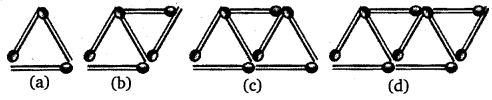Solution:
(a) Clearly, from the figure: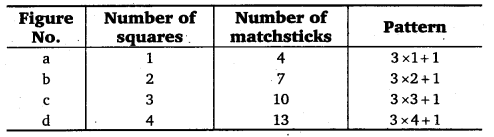Thus, rule is as under:
Number of matchsticks = 3x +1, where x is the number of squares,
(b) Clearly, from the figure :Thus, rule is as under: ,
Number of matchsticks = 2x +1, where x is the number of triangles.